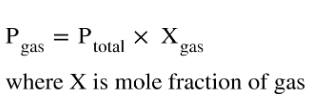# Problem: Assume that an exhaled breath of air consists of 74.7 % N2, 15.4 % O2, 3.8 % CO2, and 6.1 % water vapor.If the total pressure of the gases is 0.990 atm , calculate the partial pressure of CO2.

###### FREE Expert Solution
• From the given percentage of CO2, we can use the Dalton's Law of Partial Pressures where:• The given percentage provided will tell us the mole fraction of the CO2
86% (29 ratings)###### Problem Details

Assume that an exhaled breath of air consists of 74.7 % N2, 15.4 % O2, 3.8 % CO2, and 6.1 % water vapor.

If the total pressure of the gases is 0.990 atm , calculate the partial pressure of CO2.